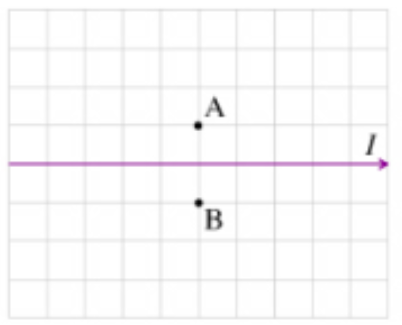# Problem: For the following two questions consider the wire shown in the figure. (Figure 1)Part AWhat is the direction of the magnetic field BA→ at Point A?a. BA→ is out of the page.b. BA→ is into the page.c. BA→ is neither out of nor into the page and BA→ ≠ 0.d. BA→ = 0

###### FREE Expert Solution

In this problem, we are required to demonstrate our ability to determine the direction of the magnetic field around straight current-carrying conductors.

88% (169 ratings)###### Problem Details

For the following two questions consider the wire shown in the figure. (Figure 1)

Part A

What is the direction of the magnetic field $\stackrel{\to }{{\mathrm{B}}_{\mathrm{A}}}$ at Point A?

a. $\stackrel{\to }{{\mathrm{B}}_{\mathrm{A}}}$ is out of the page.

b. $\stackrel{\to }{{\mathrm{B}}_{\mathrm{A}}}$ is into the page.

c. $\stackrel{\to }{{\mathrm{B}}_{\mathrm{A}}}$ is neither out of nor into the page and $\stackrel{\to }{{\mathrm{B}}_{\mathrm{A}}}$ ≠ 0.

d. $\stackrel{\to }{{\mathrm{B}}_{\mathrm{A}}}$ = 0